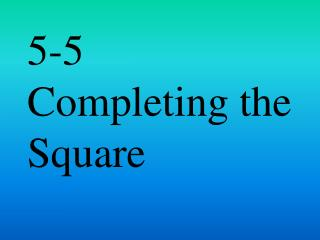DownloadDownload Presentation5-5 Completing the Square

# 5-5 Completing the Square

Télécharger la présentation## 5-5 Completing the Square

- - - - - - - - - - - - - - - - - - - - - - - - - - - E N D - - - - - - - - - - - - - - - - - - - - - - - - - - -
##### Presentation Transcript

1. 5-5 Completing the Square

2. CA STANDARD • SWBAT: • Solve quadratic equations by using the Square Root Property • Solve quadratic equations by completing the square

3. Square Root Property/Equations with Rational Roots • Solve x2 + 10x + 25 = 49 by using the Square Root Property. (x +5)2 = 49 Factor into perfect square trinomial x+5 = ±√49 Take the square root of both sides X+5 = ±7 Simplify X = -5 ± 7 Solve for variable X = -5+7 x=-5-7 Write as two equations X =2 x = -12 Solve each equation {2,-12} Answer as a solution set

4. Your Turn • Solve each equation by using the Square Root Property X2 - 12x + 36 = 25

5. Your Turn • Solve each equation by using the Square Root Property X2 – 16x + 64 = 49

6. Equation with Irrational Roots • Solve x2 – 6x + 9 = 32 by using the Square Root Property (x – 3)2 = 32 Factor into perfect square trinomial X – 3 = ±√32 Take the square root of both sides X = 3 ±√32 Solve for variable X = 3 + x = 3-√32 Write as two equations X = 3 + 4√2 x = 3 - 4√2 Simplify X ≈ 8.7 x ≈ -2.7 You can NOW use a calculator

7. Your Turn • Solve each equation by using the Square Root Property X2 + 8x + 16= 20

8. Your Turn • Solve each equation by using the Square Root Property X2 – 6x + 9 = 32

9. Completing the Square • What happens when a perfect square trinomial cannot be factored? • STEP 1: Find one half of the b • STEP 2: Square the result from STEP 1 • STEP 3: Add the result of Step 2 to the equation x2 + bx

10. Complete the Square • Find the value of c that makes x2 + 12x + c a perfect square. Then write the trinomial as a perfect square. • Find one half of the b 12/2 = 6 • Square the result 62 = 36 • Replace the c x2 + 12x + 36 • Factor (x+6)2

11. Your Turn • Find the c value that makes x2 – 14x + c a perfect square. Then write the trinomial as a perfect square.

12. Solve an Equation by Completing the Square • Solve x2 + 8x – 20 = by completing the square x2 + 8x = 20 Subtract the c value x2 + 8x + 16 = 20 + 16 Add the new c value to both sides (x + 4)2 = 36 Factor the perfect square X + 4 = ±6 Square root both sides X = -4 ±6 Solve for the variable X=-4+6 x=-4-6 Write as two equations X=2 x = -10 Simplify {-10,2} Answer as a solution set

13. Your Turn • Solve each equations by completing the square X2 – 10x + 24 = 0

14. Your Turn • Solve each equations by completing the square X2 + 10x + 9 = 0

15. Equation with a ≠ 1 • Solve 2x2 – 5x + 3 = 0 by completing the square X2-5/2x+3/2 = 0 Divide by the a coefficient X2-5/2x = -3/2 Subtract c X2-5/2x + 25/16 = -3/2 + 25/16 Add new c to both sides (x – 5/4)2 = 1/16 Factor into perfect trinomial X – 5/4 = ±1/4 Square root both sides X = 5/4 ±1/4 Solve for the variable X = 5/4 + ¼ X = 5/4 - ¼ Write as two equations X = 3/2 x = 1 Simplify

16. Your Turn • Solve each equation by completing the square 3x2 + 10x -8 = 0

17. Your Turn • Solve each equation by completing the square 3x2 – 14x + 16 = 0

18. Equation with Complex Solutions • Solve x2 + 4x + 11 = 0 by completing the square X2 + 4x = -11 Subtract c X2 + 4x + 4 = -11 + 4 Add new c to both sides (x + 2)2 = -7 Factor into perfect trinomial X + 2= ±√-7 Square root both sides X + 2 = ±i√7 Simplify square root X = -2 ± i√7 Solve for the variable {-2 + i√7 , -2 - i√7 } Answer as a solution set

19. Your Turn • Solve each equation by completing the square X2 + 2x + 2 = 0

20. Your Turn • Solve each equation by completing the square X2 - 6x + 25 = 0

21. HOMEWORK • Pg 272 • #16-23 (4) • #24-27(2) • #28-39 (6)